## Tuesday, 22 July 2008

### Bayesian DOSY: a New Approach to Diffusion Data Processing

Yesterday I blogged about basic concepts on DOSY NMR. From an experimental stand point, the pulse sequences are not very complex, being the Pulse Field Gradient Stimulated Echo (PFGSE) experiment proposed by Tanner one of the basic pulse sequences used to determine diffusion by NMR. This pulse sequence can be considered as a building block for a number of extended pulse sequences designed to minimize some sources of artefacts caused by thermal convection currents in the sample, background gradients, radiation damping, zero order coherences in strongly coupled spin systems, etc. Other effects such as J-modulation and Cross-Relaxation have also been considered.

The tricky part comes in the mathematical DOSY transformation. As I mentioned earlier, there exists different approaches, each of them with their own strengths and weaknesses. Today I would like to introduce a new method for DOSY transformation based on Bayesian Theory which has been implemented in our software Mnova as a result of our collaboration with Stan Sykora. We call this new algorithm BDT (Bayesian DOSY Transform)

A formal description of the Bayesian theory and its particular implementation for DOSY transformation is beyond this blog entry, but we are currently writing an article which will give all the details of the method as implemented in Mnova.
In short, this Bayesian approach assigns an a-priori probability (this is the key word in Bayesian context) to the elements in a space defined by the entities to be estimated. In this context, an entity is the pair (f,d) where f is frequency and d is a mono-diffusion coefficient. Actually, it should be (f,d,w) where w is a weight (intensity), but this weight is factored out by finding its optimal value (since the dependence is linear, this can be done explicitly). So we are actually finding the a-posteriori probability for the sentence there is a component - no matter how intense - at f that has such and such d. Next, a final normalization process modifies the probability still further (composite probability) by looking at the intensity in the spectrum at location f (this would be real probability if the spectrum were normalized to 1).

Stan Sykora will be presenting the mathematical background and physical insights necessary to understand this new method as well as some real life examples processed with Mnova in the GIDRM conference to be held in Bressanone (Brixen) next September.

So how does the algorithm perform? We first tested the algorithm by simulating with Matlab a diffusion experiment with 2 synthetic peaks with frequencies of 100 and 200 Hz and diffusion coefficients of 1 and 0.01 (dimensionless).Application of BDT yields the following DOSY spectrum: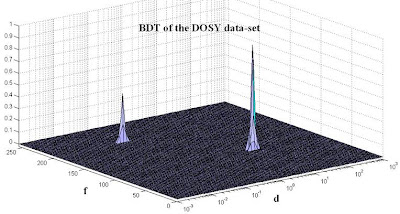The synthetic DOSY spectrum was changed in order to include artificial noise and different degrees of peak overlap and diffusion distances. We’ve found the performance of the algorithm to be excellent in all the cases analyzed.

Next we implemented the algorithm in Mnova and we applied it with a sample of an aqueous solution of potassium N-methyl-N-oleoltaurate (a surfactant) with TSP at 23 C (The original Varian FID file has been obtained from the VARIAN NMR USER GROUP LIBRARY which was submitted by Brian Antalek as a sample for this DECRA algorithm). This is the original raw data after automatic Fourier Transform, phase and baseline correction in Mnova:After applying BDT, we obtain a DOSY spectrum in which the three components are clearly well resolved in the diffusion dimension and the absolute values are in accordance with the expected values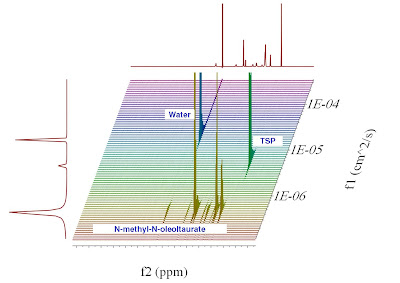Below you can see the DOSY spectrum of a mixture of Caffeine + 2-EthoxyEthanol + Water acquired in a Bruker instrument by my friend Andy Soper.Main properties of the algorithm

Compared to other approaches, we believe that this Bayesian method implemented in Mnova appears extremely promising. It automatically avoids having exact, unnatural zeros anywhere in the resulting DOSY map since every point of the 2D map has a well defined value of statistical congruence with the data. Moreover, the BDT maps show ‘normal’ line widths in the f-direction, correctly positioned and resolved peaks in the d-direction and quantitatively correct horizontal and vertical projections – a combination difficult to achieve by any other means.
The approach can be easily extended to non-exponential cases arising from overlapping lines and, like all Bayesian methods, incorporate additional information available from other sources (a-priori knowledge). Likewise, it is possible to place a statistical premium on alignment of spectral peaks along horizontal lines in the [f,d] plot.

Do you want to try it out yourself?

The algorithm is readily available in the alpha stage in Mnova. From this post, I would like to offer this special version to anyone interested in trying the BDT algorithm with his own data sets. I will very much appreciate any feedback from you.
Just write to me at the email address below and I will give you the instructions on how to get the software.

## Sunday, 20 July 2008

### DOSY NMR

NMR diffusion experiments provide a way to separate the different compounds in a mixture based on the differing translation diffusion coefficients (and therefore differences in the size and shape of the molecule, as well as physical properties of the surrounding environment such as viscosity, temperature, etc) of each chemical species in solution. In a certain way, it can be regarded as a special chromatographic method for physical component separation, but unlike those techniques, it does not require any particular sample preparation or chromatographic method optimization and maintains the innate chemical environment of the sample during analysis.
The measurement of diffusion is carried out by observing the attenuation of the NMR signals during a pulsed field gradient experiment. The degree of attenuation is a function of the magnetic gradient pulse amplitude (G) and occurs at a rate proportional to the diffusion coefficient (D) of the molecule. Assuming that a line at a given (fixed) chemical shift f belongs to a single sample component A with a diffusion constant DA, we have

(1) S(f,z) = SA(f) exp(-DAZ)

where SA(f) is the spectral intensity of component A in zero gradient (‘normal’ spectrum of A), DA is its diffusion coefficient and Z encodes de different gradient amplitudes used in the experiment.

Depending on the type of experiment, there are various formulae for Z in terms of the amplitude G of the applied gradient and one or more timing parameters such as
Δ (time between two pulse gradients, related to echo time) and δ (gradient pulse width). In the original Tanner-Stejskal method using two rectangular gradient pulses, for example,

(2) Z =γ2G2δ2(Δ-δ/3)

Eq. (2) holds strictly for simple PFG-NMR experiments, and is modified slightly to accommodate more complicated pulse sequences.

In practice, a series of NMR diffusion spectra are acquired as a function of the gradient strength. For example, the figure below shows the results of a series of 1H-NMR diffusion experiments for a mixture containing caffeine, 2-Ethoxyethanol and water.It can be observed that the intensities of the resonances follow an exponential decay. The slope of this decay is proportional to the diffusion coefficient according to equation (1). All signals corresponding to the same molecular species will decay at the same rate. For example, peaks corresponding to water decay faster than the peaks of caffeine and 2-Ethoxyethanol

The DOSY transformation

As far as data processing of raw PFG-NMR spectra is concerned, the goal is to transform the NxM data matrix S into an NxR matrix (2D DOSY spectrum) as follows: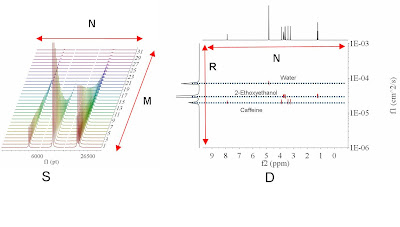The horizontal axis of the DOSY map D is identical to that of S and encodes the chemical shift of the nucleus observed (general 1H). The vertical dimension, however, encodes the diffusion constant D. This is termed Diffusion Ordered Spectroscopy (DOSY) NMR.
In the ideal case of non-overlapping component lines and no chemical exchange, the 2D peaks align themselves along horizontal lines, each corresponding to one sample component (molecule).

The horizontal cut along such a line should show that component’s ‘normal’ spectrum. Vertical cuts show the diffusion peaks at positions defining the corresponding diffusion constants.

The mapping S=>D will be henceforth called the DOSY transformation. This transformation is, unfortunately, far from straightforward. Practical implementations include mono and biexponential fitting, Maximum Entropy, and multivariate methods such as DECRA.

Recently, Mathias Nilsson and Gareth A. Morris have proposed the so-called ‘Speedy Component Resolution’ (Anal. Chem., 2008, 80, 3777–3782) as an improved variation of the Component Resolved (CORE) method (J. Phys. Chem, 1996, 100, 8180). This is a multivariate-based method and the examples used in the article show an excellent performance of the algorithm.

Following a different approach, we have recently developed a brand new method for DOSY processing which has been included in Mnova. I will leave the details (and how to get the program to try it out) for the next post. In the meantime, should you be interested, just drop me a line.

## Tuesday, 1 July 2008

### Sensitivity Enhancement for free?

In my previous post I discussed about NMR FID/spectra having two channels, the Real and Imaginary parts and that in general, only the Real part is displayed. In fact, we could use the term Stereo-FID in the same fashion as we use Stereo-Sound (remember that NMR spectra span audio frequencies).
If we think about these stereo signals as coming from two receiver coils in quadrature and assuming simultaneous detection, we could formulate the following scenario:

It’s a common practise to acquire several (hundred or thousand) FIDs which are then added together (signal averaging). Because NMR responses build up in proportion to the number of signals recorded, N, whilst the noise varies randomly from one measurement to the next and thus, adds up more slowly, as sqrt(N), there is an overall improvement in sensitivity of sqrt(N).
If the noise in the Real and Imaginary channels were totally independent, it should be possible to add to the Real channel the Imaginary channel (after a 90º phase shift to make it phase coherent with regard to the Real part) so that we could achieve a further improvement of sensitive of sqrt(2)!. Would this be possible? This will be the subject of this post.

Of course, this ‘trick’ would only work if the noise in the two channels were totally independent. In the NMR field it is assumed that the experimental noise is stationary and white (i.e. the correlation between two consecutive points is zero). But what about the correlation between the noise in the two different channels? Are they correlated? This is very easy to analyze experimentally with Mnova by writing a very simple script which calculates the Correlation Coefficient (Pearson). For example, consider the spectrum below. We could use this script to calculate the Pearson Correlation Coefficient using the points between 5000 & 10000 which is a signal free regionAs shown in the figure below, the correlation coefficient between the noise in both channels is almost zero. You can repeat this operation with different spectra and you will arrive to similar results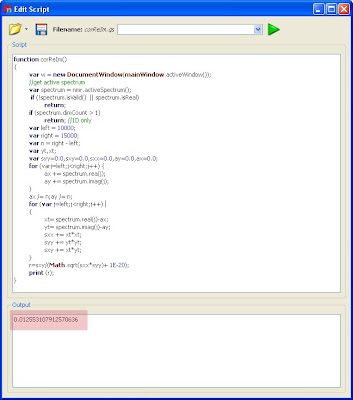So it appears as if the noise in both channels is statistically uncorrelated, something which should be intuitively expected as the 2 acquisition channels are orthogonal. Does this mean that the noise in both channels is independent?
We can make a very simple experiment: We could phase a spectrum in order to make the real part perfectly in phase and then change the phase of the imaginary part in such a way that the peaks in both channels become perfectly phase-coherent. Next, both channels can be added so that the signals will increase 2 fold. On the other hand, if the noise in both channels were phase-incoherent, it will increase more slowly and therefore the overall S/N should increase by sqrt(2).

We can carry out this experiment very easily by applying a 90º phase shift into the imaginary channel and then summing up both channels. This is done with the following script:Before applying this script, we need to calculate the SNR of the original spectrum. We can use the central peak of the Chloroform multiplet as a reference peak to estimate the SNR as depicted in the figure below: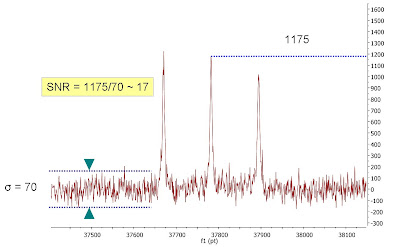Next, we can apply the script above (sumReImPhased) to apply a 90º phase shift to the imaginary part followed by the addition of the imaginary channel to the real one. If the SNR of this new spectrum is calculated we get the following result:We can appreciate that the Chloroform peak is now twice the height, but the standard deviation has also increased two-fold, so the SNR remains constant. What a disappointment!

Conclusions:

We have first found out that the noise in the Real and Imaginary channels is statistically uncorrelated. However, this does not mean that they are independent. Just as sin(x) and cos(x) functions are orthogonal "uncorrelated", but not independent (sin^2(x) = 1 – cos^2(x)).
And we have shown that noise in the Re and Im channels is certainly not independent: the SNR does not improve at all.
The essence is that if the spectrometer has just one coil (which is, to the best of my knowledge, always the case in spectroscopy), then the noise in the two orthogonal channels would not be independent and thus the sqrt(2) sensitivity enhancement is not possible. It will be necessary to have two separate coils (and two receivers) in quadrature to have uncorrelated real and imaginary noise. Why is this not possible? I don’t really know, most likely because of lack of space …

References:

Experimental Noise in Data Acquisition and Evaluation III. Exponential Multiplication, Discrete Sampling, and Truncation Effects in FT Spectroscopy. Dr. S. Sýkora.